### DIMENSION PART 2

by Mike Mongan

In the first part, we showed that a regular, two dimensional polygon can define a regular, three dimensional polyhedron in its projection. We started with an equilateral triangle and wound up with a dual tetrahedron which in turn defined an octahedron and a cube (hexahedron). We discussed this process as an intervention by time.

Now, an exercise. A soda straw will work for this, a long thin piece of paper will also. Flatten the straw over the edge of a table. Tie it into a simple overhand knot. As you pull the knot tight, be careful not to crush the flat part. It should look like figure 1 . Hold it up to the light and notice the center. It is a regular pentagon. The pentagon has many remarkable properties. We will look at some of them in this article. As you have just seen it is a natural result of a simple knot in a flat surface.Figure 1 A Knot in a Soda Straw

If we take two pentagons, keep their centers common and rotate one so that its vertices are equally distant from the other, we will have defined a regular decagon - a ten sided figure. Together with all of its chords, it is shown in figure 2. We will designate the cords in this manner: a line connecting two adjacent vertices of a "tenagon" will be called A¢10, a line connecting every other vertex is called B¢10, a line connecting every third vertex is called C¢10 and so on. If we were dealing with another figure, like the pentagon, then we would use A¢5 to indicate the first chord, etc. (A cent sign in this context is shorthand for chord.)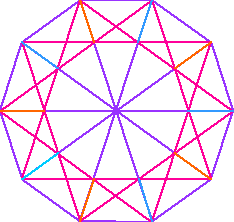Figure 2 Decagon with Chords

Notice in the pattern of the C¢10 chords, we identify the vertices of another decagon inside the first. Use this to create two pentagons. Take one and connect each of its vertices radially outwards to a vertex of the outer figure. Notice how each side seems to have its own squished pentagon. Do the same with the other pentagon. This is a top view of a dodecahedron.Figure 3 Top View of a Dodecahedron

Ordinary drafting methods project this view into the two additional views shown in figure 4. For those unfamiliar with it, the regular dodecahedron is one of the platonic solids. All of its edges are of the same length and all angles are equal. There are thirty edges, twenty vertices and twelve faces, each a regular pentagon. Not bad for a knot in a soda straw! The construction lines are not shown here, but this drawing was done on a 2-d cad program with standard drafting techniques. It could be done with compass and straight edge. A more practical manual method would be with compass, protractor and two triangles.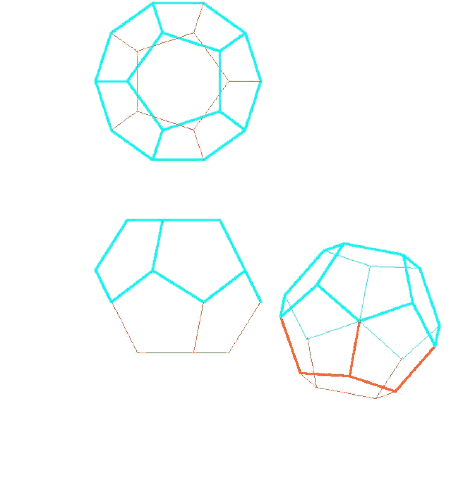Figure 4 Projected Views of a Dodecahedron

Since all of the edges are equal and all of the faces are regular, then all of the B¢5 chords of the faces are equal too. Selecting these in the manner shown reveals our old friend the cube. This is not the only cube we could have defined. Each face has 5 each B¢5 chords for a total of sixty. Each cube has twelve edges. So a regular dodecahedron will define five cubes. This is one of the possibles.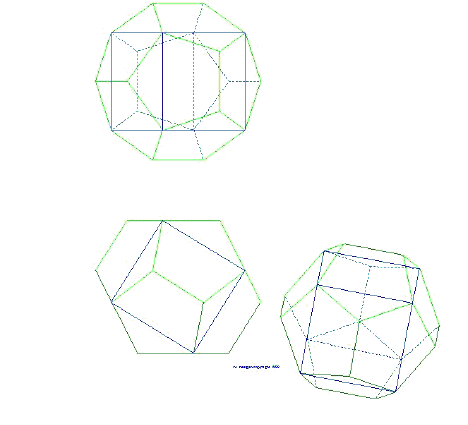Figure 5 Cube in the Dodecahedron

There is another way to look this figure, not as a dodecahedron with a cube at the center. Look at it as a cube with six roofs on it - like the roofs of houses. Instead of just being on the top, They are on all the sides. Each is exactly the same, except for its orientation. Figure 6 shows a cube house with one roof. Figure 7 shows an exploded view of all of them. Figure 8 takes us back to the dodecahedron.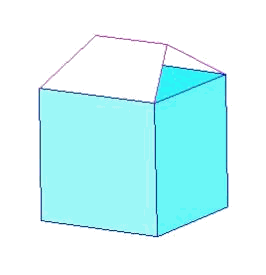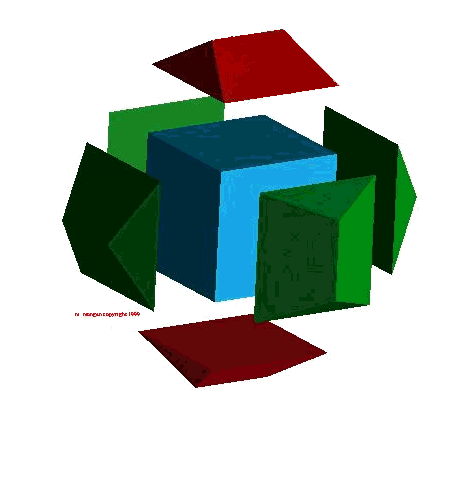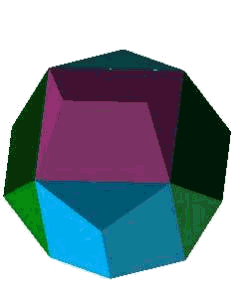Figure 6 Figure 7 Figure 8 Cube House with Dodec Roof Exploded View Dodecahedron

This roof can be thought of as the bridge figure between the cube and the dodecahedron. As such, it has the properties of both. I started this article with a mention of the special properties of the pentagon. It is time to take a closer look at them. To do that we must first take a dive into ratios. A ratio is the relationship between two like entities. Ratios are normally expressed as two numbers with a colon between them as 2:3. It is similar to a fraction but also has the properties of the reciprocal of that fraction. It is as much 3/2 as it is 2/3. A fraction puts a direction to the ratio. A fraction indicates division. Division asks how much bottomness is contained in the top. Ok, I know my terminology is sloppy, so it's how much denominatorness is contained in the numerator. That's why you can't divide by zero. You're asking how much nothing (zeroness) there is in something. In the converse, where zero is the numerator, you're asking how much somethingness there is in nothing.

In the case of the pentagon that ratio has been given a special name - the golden ratio or the golden section. It is often denoted by the greek letter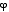. Mankind has been aware of the golden ratio for untold millennia. This ratio has been used as the measure of perfection and beauty in art and architecture for ages. A lot has been written on it already, so let's not get too far afield. Mathematically it is expressed as: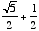. This is approximately equal to 1.618033989... Just to tempt you with a couple of its properties:+ 1 =x- 1 = 1/They go on and on. The easy way to draw it is shown in figure 9. The diagonal of half a square is rotated down to the base of a second square. Both squares have sides equal to 1. The Pythagorean theorem gives you the rest.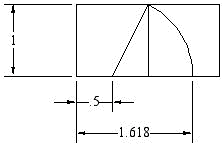Figure 9 Square Phi

The easy way to make it is with the simple overhand knot you made at the beginning. Figure 10 illustrates that when A¢5 = 1, B¢5 =.Figure 10 Pentagonagram

Ratio is also the basis of music. When you watch a guitar player, notice how the fingers push the strings down at different places or frets on the neck to create higher notes. This is because the strings vibrates faster when they are shortened. If a string is fretted at half its length, it is one octave higher than if it plucked at its base length. In classical times the notes of the scale were based upon strict geometric ratios. The modern musician tunes slightly off these ratios for aesthetic reasons. But whatever we choose to be our defining note, it is purely arbitrary. If we say that the base note is A at 440 cycles per second it is just a matter of choice. The same is true with our measures of time. Our ultimate measure of time these days is an atomic clock. But like all measures of time, it is an arbitrary standard for seconds,minutes and hours.

A ratio requires at least two entities. The golden section - or any ratio - can be expressed as a dividing point along a one dimensional line length. The projection of that ratio into a two dimensional space results in a plane figure. The projection of it into three dimensions results in a solid figure. Figure 11 was used to create the three dimensional illustrations above. It is essentially a ratio. The two opposing interior pentagons in figure 3 are the starting spot. The shoulders of one determine the edge length of the cube. The opposite pentagon is projected into a prism whose height is determined by diagonal of the cube's face. If the entire decagon from figure 2 were shown and projected into a decadrum, the other corners of the cube would fall into place as in the drawings. This prism determines two faces of the dodecahedron, it would take five others to complete the figure.Figure 11 Pentagonal Prism and Cube